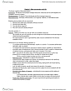ECO 1102 Study Guide - Final Guide: Gdp Deflator, Nominal Interest Rate, Physical Capital

192 views2 pagessage123is and 43 others unlocked46
Verified Note
46 documents

Document Summary

Components of gdp: gdp (y)= consumption (c) + investment (i)+ government purchases (g)+ net. Exports (nx: note: net exports= exports- imports. The gdp deflator: gdp deflator= (nominal gdp/ real gdp) x 100. Inflation rate in current year= [(gdp deflator in current year- gdp deflator in previous year)/ gdp deflator in previous year] x 100. Consumer price index (cpi): cpi= (cost of basket of goods in current year/ cost of same basket in base year) x 100. Inflation rate in current year= [(cpi in current year- cpi in previous year)/ cpi in previous year] x 100. Comparing dollar figures from different times: price in todays dollars= (price in base year"s dollars) x (cpi today/ cpi base year) Real interest rate= nominal interest rate- inflation rate. Factors of productivity: (1) physical capital per worker (k) (2) quantity of labour (l) (3) human capital per worker (h) (4) natural resources per worker (n) (5) technical knowledge (a)